# A* Algorithm (Graph Traversal and Path Search Algorithm)

## Introduction

Pathfinding has been used in various computer science fields. In video games, pathfinding can be used to move objects from their initial place to their destination in the shortest route. As video games develop, pathfinding is becoming increasingly popular in various games, such as tile-based or map-based. One of the most popular pathfinding algorithms is the A-Star algorithm.

The shortest path problem is the problem that finds the minimum distance or pathway between nodes or vertices in a graph (for example, in a road network). Many algorithms solve the shortest path problem. Dijkstra’s algorithm is one form of the greedy algorithm. A heuristic is a method designed for solving a problem more quickly. This is achieved by trading accuracy, optimality, completeness, or precision for speed. The A-star algorithm is an algorithm that finds a path in a graph from a given initial node to a destination node. It used a “heuristic estimate” h(n) that estimates the best route through that node. It visits the nodes in order of this heuristic value.

The A-star algorithm is a searching algorithm used to find the shortest path between an initial and a final point. The algorithm is optimal and complete as it searches for shorter paths first. An optimal algorithm finds the least-cost outcome for a problem, while a complete algorithm finds all the possible outcomes.

For example, in a square grid, many obstacles it is used to calculate the shortest distance between the source(orange) and the destination(cyan).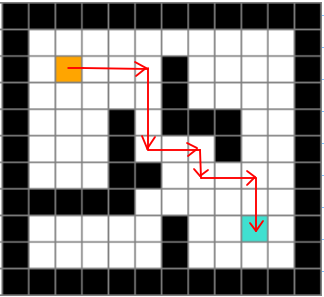### Concept of A-star algorithm

The A-star algorithm calculates the cost to all its neighboring nodes and chooses the minimum cost node. This process is repeated until no new nodes can be selected and all the paths are traversed. Then, we consider the best path. Let f(n) represents the final cost, which is denoted as: f(n) = g(n) + h(n), where:

• g(n) = cost of traversing from one node to another.
• h(n) = heuristic approximation of the node’s value.

### Calculation of Heurestic

There are two ways to calculate h. We can either calculate the exact value of h or approximate the value of h using some heuristics. The first method is time-consuming, whereas the second method is less time-consuming and inaccurate.

#### Exact Heuristics calculation

Following are some methods to calculate the exact value of h:

1. Pre-compute the distance between each pair of cells before running the A-star Algorithm.
2. If there are no blocked cells(obstacles), we can find the exact value of h without any pre-computation using the Euclidean Distance.

#### Approximate Heuristics calculation

Following are some approximate heuristics to calculate h.

Euclidean Distance

1. The distance formula is the distance between the current node and the destination node.
2. h = sqrt ( (node.x-goal.x)² + (node.y -goal.y)² )
3. This heuristic can be used when we can move in any direction.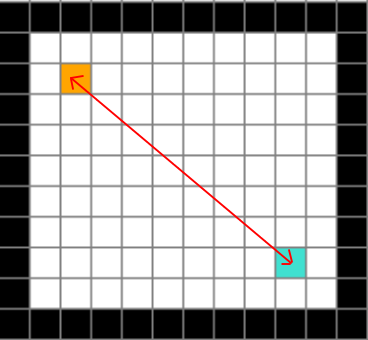Manhattan Distance

1. It is the sum of absolute values of differences in the current cell’s x and y coordinates and the goal’s x and y coordinates.
2. h = abs (node.x-goal.x) + abs (node.y-goal.y)
3. This heuristic can be used when we can move in 4 directions only(up, down, left, right) in a 2d grid.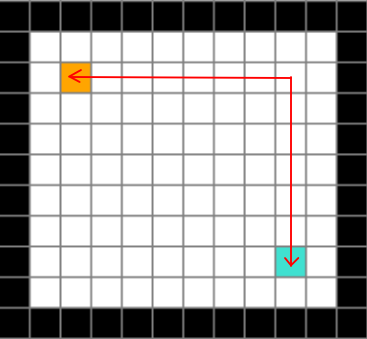Diagonal Distance

1. This is used for 8-way movement when the diagonal direction cost differs from the non-diagonal cost.
2. h = costD * dMin + costN * (dMax -dMin), where costD = cost of diagonal movement = sqrt(2) * costN in our case. Also dMax = max(abs(node.x-goal.x), abs(node.y -goal.y)) and dMin = min(abs(node.x-goal.x), abs(node.y -goal.y)).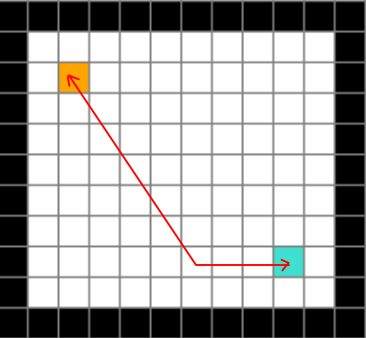### How does the A-star algorithm work?

Consider the following graph, N1 is the source, and N4 is the destination.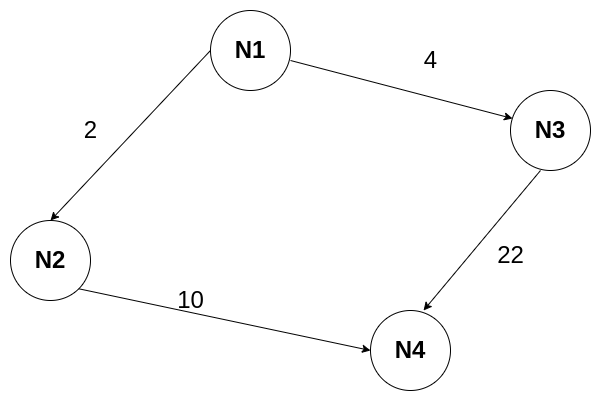The start is at the source N1, which has g = 0 and some initial heuristic value h. Therefore, f(N1) = g(N1) + h(N1) => f(N1) = 0 + 10 = 10. Next, consider the path to the neighbouring vertices:

• f(N1->N2) = g(N1->N2) + h(N1->N2) = 2 + 5 = 7
• f(N1->N3) = g(N1->N3) + h(N1->N3) = 4 + 6 = 10

Now consider the path to the destination:

• f(N1->N2->N4) = g(N1->N2->N4) + h(N1->N2->N4) = 10 + 3 = 13
• f(N1->N3->N4) = g(N1->N3->N4) + h(N1->N3->N4) = 22 + 5 = 27

We can see that choosing node N2 from N1 gives the best path. Note that Dijkstra is a special case of A* Search Algorithm, where h = 0 for all nodes. It is just like Dijkstra’s algorithm but the only difference is that A-star tries to look for a better path by using a heuristic function, prioritizing nodes that are better than others while Dijkstra explores all possible ways.

Thus in the A-star algorithm, instead of checking for g(n) for the current node like in Djisktra’s, we check for f(n) = g(n) + h(n) for the current node. Here g(n) = distance from source to parent + distance from parent to the current node, and h(n) is the heuristic function explained above.

Now in the A-star algorithm, we do not visit all the nodes. We start from the source node, and then check all the neighbors of this node, then for each neighbor add its g value (distance from parent to this neighbor) to the g value of the parent node, then add its h value to this, giving the f value (f = g + h).

Then add all these neighbors to a priority queue according to their f values. We use a priority queue so that we can get the smallest value in O(logn) time and we can insert values in O(logn) time. The nodes in the priority queue are now OPEN to calculate, and the source node is CLOSED to calculate. (Initially, the source node is OPEN, and the CLOSED list is empty).

Next, we go to the node with the smallest f value (if the destination is not reached already) and then visit it only if this node does not already have a shorter path available (smaller f value, maintained in the list for each node, which is initially infinity for all nodes except source). If there is already a shorter node to this node, then the current path is not the shortest, and hence we do not expand its neighbors, and we can make the node CLOSED and return to the next shortest path in the priority queue. We repeat this till we reach the destination node. The moment we reach the goal, it is the shortest path and a guaranteed one if the heuristic is consistent.

### Pseudocode of A-star algorithm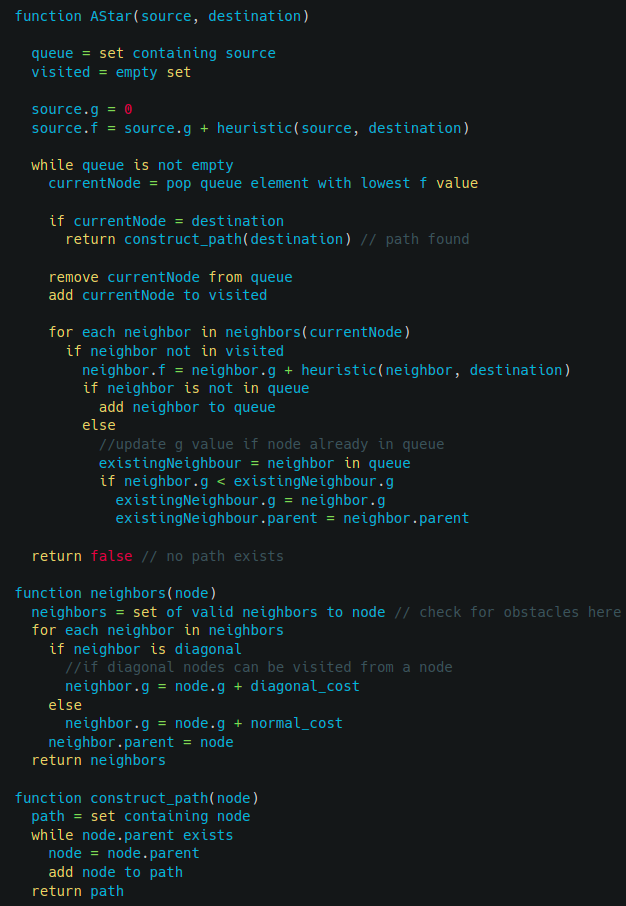Time and Space Complexity

In the worst case, the A-star algorithm travels all the edges to reach the destination from the source. So the worse case time complexity is O(E), where E is the number of edges in the graph. In the worse case, we can have all the edges inside the open list, so the required extra space in the worst case is O(V), where V is the total number of vertices.

Enjoy learning, Enjoy algorithms!

☆ 16-Week Live DSA Course
☆ 10-Week Live DSA Course